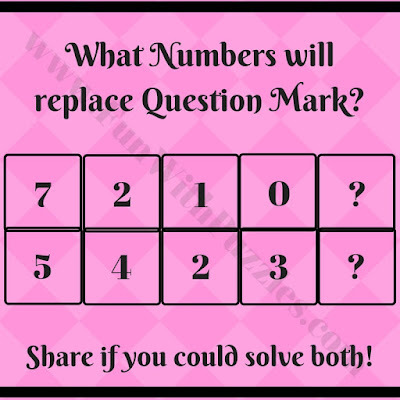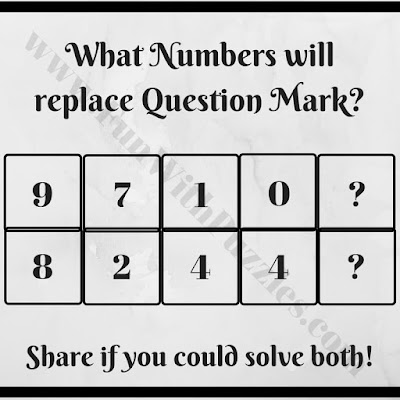## Thursday, July 30, 2020

When it comes to Fun Brain Teasers, Math Brain Teasers are very interesting brain teasers in which puzzle questions are based upon mathematical operations. In such Maths Mind Games, few numbers are given in some sequence or around a particular shape. These numbers relate to each other with some maths formula or mathematical operations. One has to find this hidden relationship among these numbers and then solve it for the missing numbers. Today's mind games are based upon two parallel number sequences.
In these Maths Mind Games, two parallel sequences of numbers are given. These numbers follow some sequential patterns or some logical or mathematical relationship. Find these patterns in these puzzles and find the missing numbers at the end which will replace the question marks.
Answers and explanations of these Math Mind Games are given at the end of this post. Utilize these answers to only validate your solutions. In case you find some different solution to any of these Math Mind Game Puzzle Question, do write about it in the comments section along with your reasoning to solve these puzzles. It will be ingesting to find some different logic to solve these Maths Mind Games. :)1. Can you solve it for the missing number?2. Can you find the missing numbers?3. Find the missing numbers in this puzzle4. Can you find the missing numbers?5. Can you solve this puzzle to find missing numbers?

These tricky Maths Mind Game Brain Teasers tested both your Mathematical skills as well as Logical Reasoning. Do try out below mentioned puzzles and brain teasers to further test your Maths and Logical skills.

## List of Puzzles and Brain Teasers

1.What are the logic for the answer

1.Please do post your answers in case your answers differs. It will be interesting to know the different logic to solve these puzzles. Do let us know for which question you are looking for the explanation, we will post the logic for that question soon?

2.We have now added logical explanation for each of the answer. Do post your comments in case you got different reasoning for any of these brain teasers.

2.What is the answer for the thumnail

1.Thumbnail picture is just snapshot. However there are similar missing number puzzles here on this website which you can check it out.

2.How to know the logical answer..its very hard

3.Check the link given at the end of the puzzle images. It will lead you to the puzzle video containing the answers and explanations of these puzzles.
Do let me know if something is not clear?

3.1.You can check the answers to all the puzzles at the link given in the post after the images. Please let me know if there is some problem regarding these answers?

4.I have one qus anyone plz solve and give answer as well as explanation.
Here the qus is:
2 7 6 0 ?
8 4 4 4 ?
Find the missing number ?

1.Here is the ans:
col1 & col2 gives you col3
(8x7) + (4x2) = 64 or 6,4; here we add the
multiples
col2 & col3 gives you col4
(7x4) - (6x4) = 04 or 0,4; here we substract
the multiples. we alternate adding and
substracting the multiples. So, next add
col3 & col4 gives col 5
(6x4) + (4x0) = 24 or 2,4 is the answer
suppose there was a col6, we substract
the multiples; for example
(4x2) - (4x0) = 08 or 0,8

5.1.The answer link to these tricky maths mind game brain teasers is given in the post. Check it out and let me know if something is not clear?

6.Ajith Kumar, here is the ans:
col1 & col2 gives you col3
(8x7) + (4x2) = 64 or 6,4; here we add the
multiples
col2 & col3 gives you col4
(7x4) - (6x4) = 04 or 0,4; here we substract
the multiples. we alternate adding and
substracting the multiples. So, next add
col3 & col4 gives col 5
(6x4) + (4x0) = 24 or 2,4 is the answer
suppose there was a col6, we substract
the multiples; for example
(4x2) - (4x0) = 08 or 0,8

A comment doesn't cost a thing. Please drop a comment below to boost the author's morale.